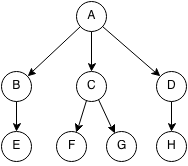## Introduction

Trees are data structures that follow a hierarchy, each node has exactly one or zero parents and each node has children. Trees are recursive structures meaning that each child of a tree is also a tree. A tree within another tree is called a subtree.

A child is a node that is below another node.

A parent is a node that is above another node.

The element at the top of the tree with no parents is called a root. The node at the bottom of the tree with no children is called a leaf.

Each node can hold different kinds of information depending on the tree. A node can hold the children it has, the parent it has, a key associated with the node and a value associated with the node.• A is the root of the tree and E,F,G,H are leaves.
• The parent of E is B.
• C,F,G is a subtree of the original tree.

## Binary Tree

A binary tree is a tree where every node has at max two children.